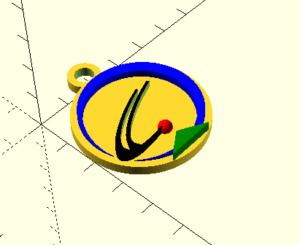# 3D Printing

Laurent software Tutorials and 3D models
Tutorials

## 4. Good practice

Like in all programing languages there is good practice you must do to ensure that everyone can understand your code and also to be easier to reread. In openSCAD it’s not so essential to do some of this good practice because the code is quite easy to understand but I definitely recommend you to do it.

### a. Commentary

To do a commentary in openSCAD you can use // to comment 1 line or /* at the start and */ at end to comment an entire block of code.

```//this is a commentary

Cube(10) //this is a cube```
```/*

hull() {

cube([20,20,1],center=true);

translate([0,0,20]) cylinder(1,8,8);

}*/```

Commentary can be used to document your code or to comment code you don’t need for the moment.

### b. Indentation

Indentation is when you do space in your code. Indentation is essential to have a clear code:

```hull() {

……………….cube([20,20,1],center=true);

……………….translate([0,0,20]) cylinder(1,8,8);

}

```
```scale([1.3,0.9,1])

linear_extrude(height=2)

difference() {

difference() {

circle(r=18);

union() {

translate([0,2,0]) circle(r=14);

translate([-60,0,0]) square([60,60]);

translate([-40,-40,0]) rotate([0,0,55]) square([60,60]);

translate([-9,-14.2,0]) rotate([0,0,-20]) square([7,3]);

}

}

}```

### c. Global variables

Global variables are in programming when you give at the beginning of the program a value that can be reused everywhere in your code. To do this you need to use the “=” operator with the syntax: name = value; .

Example:

```my_height = 20;

cylinder(my_height,19,19);